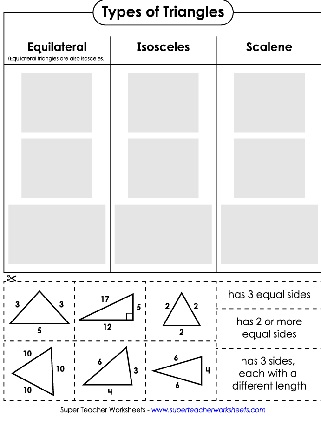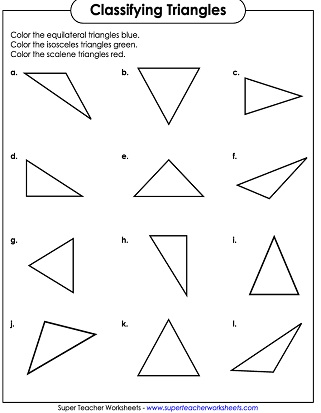# Classifying Triangles / Types of Triangles

On these printable worksheets, students will practice identifying and classifying triangles. On some worksheets, they will sort triangles by angle, identifying Acute, Right, and Obtuse triangles. On others they will sort by length of sides, identifying Scalene, Isosceles, and Equilateral triangles.## Classifying by Side Lengths:Equilateral, Scalene, Isosceles

Identify each triangle type by the lengths of its sides.
Determine which of the pictured triangles are isosceles, equilateral, scalene, and right.
Cut out the triangle pictures and definitions. Sort them on the chart and glue them to the paper.
Color the scalene triangles red, the isosceles triangles green, and the equilateral triangles blue.

## Classifying by Angles:Acute, Obtuse, Right

Cut out the triangles at the bottom of the page. Sort them into three groups. Glue them down onto the page.
Color right triangles red, obtuse blue, and acute green.
Angle Worksheets

Print worksheets on classifying, measuring, and drawing angles.

Areas of Triangles

Geometry Worksheets

These printables cover a wide-variety of topics, including polygons, circles, area, perimeter, coordinate planes, ordered pairs, lines, points, tessellation, and symmetry.

## Sample Worksheet ImagesMy Account
Site Information